It is the optimal solution table that has to be placed in a correct manner, interpreted properly to get solution values of primal.

However, it is important to understand the 2 properties of primal solution:

1. Primal Dual Property Part 1:

In this case, first it is found in case of both primal and dual function, whether a feasible solution exists. If that is the case then those optimal solutions that have the same objective values are similar. There is a peripheral relation that is created which provides no solution to problems with unbound solution.

1. Primal Dual Property Part 2:

The major problem is getting optimal values for decision variables. Here an optimal table is consulted and it is read from the point (0), wherein another problem is also considered.

Post these properties, it is important that certain steps are taken into consideration.

Step 1: In dual problems there are specifically slack-surplus variables that are associated with variables at a basic level of primal in case of optimal solution. So, slack-surplus variables have to be identified in dual problems as well.

Step 2: In case of reading optimal value of primal variables that are basic in nature, can be read directly from index row elements that are corresponding to slack-surplus variables associated with columns.

Step 3: In case of primal, the value of slack variables that are available have to be taken on taking from index row placed under non-basic variables with a sign change

Step 4: In both primal and dual problems, value of objective function remains the same.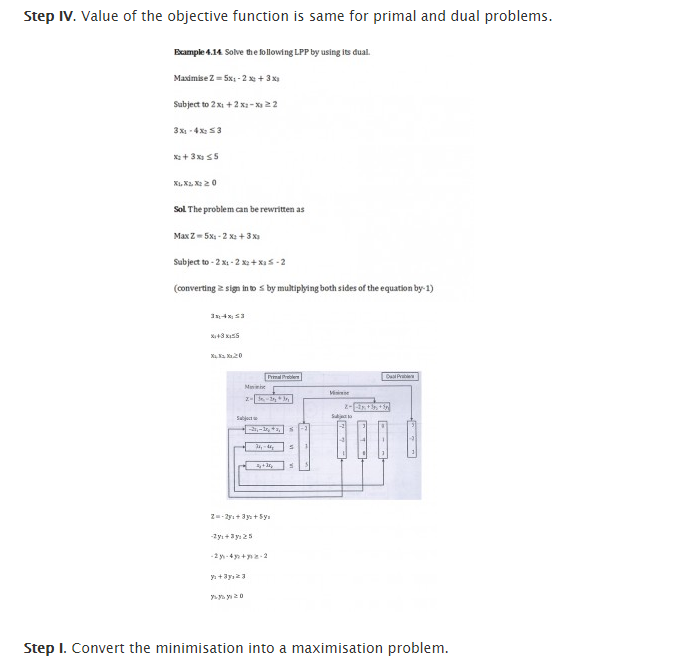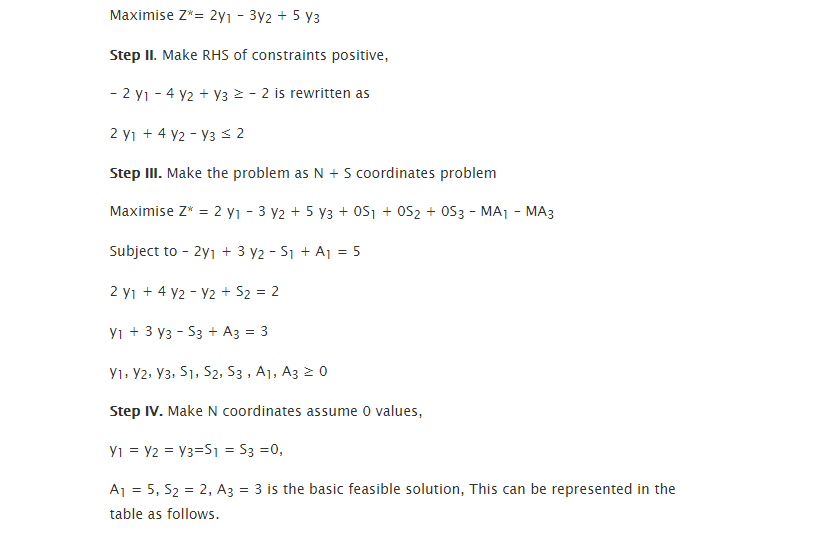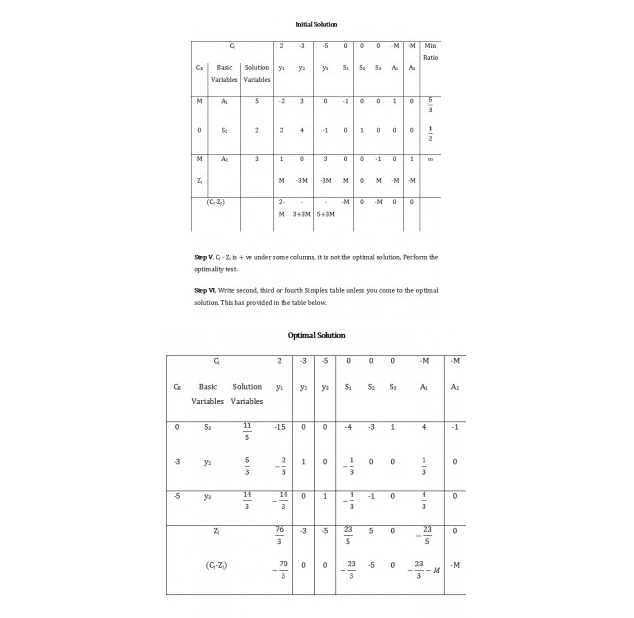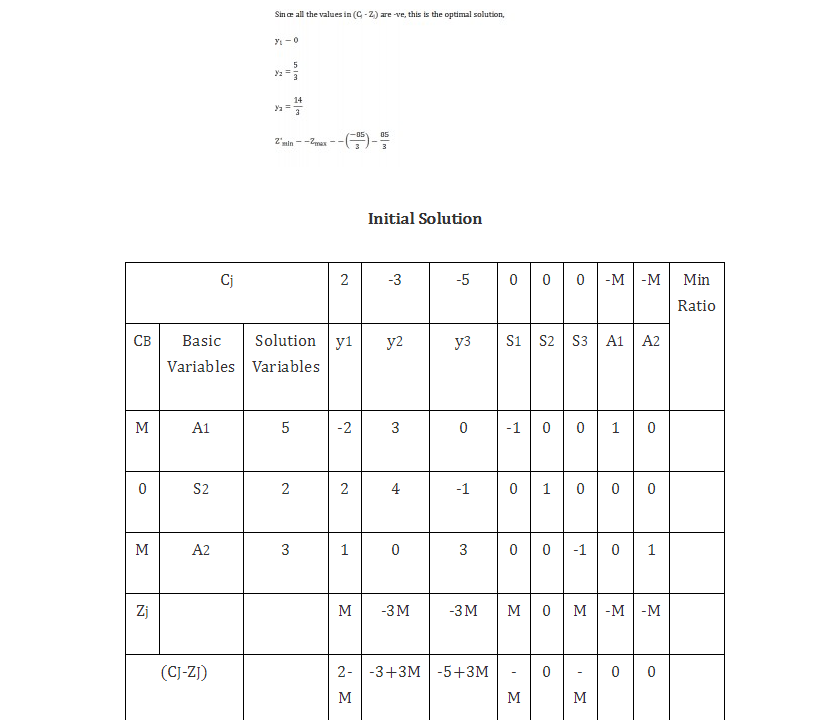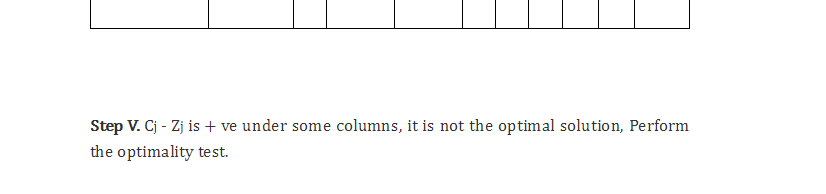### Customer Reviews

My Homework Help
Rated 5.0 out of 5 based on 510 customer reviews at